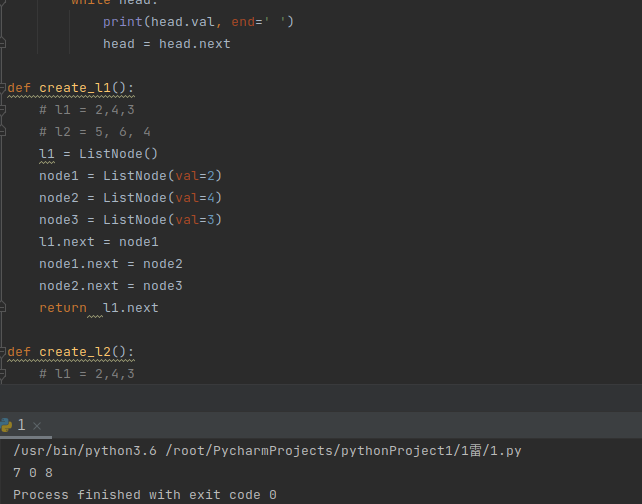signed

QiShunwang

“诚信为本、客户至上”

# python面向对象编程介绍及三大特性

2021/6/24 21:51:37   来源：

## 一面向对象编程的介绍：

“面向过程”(Procedure Oriented)是一种以过程为中心的编程思想。分析出解决问题所需要的步骤，然后用函数把这些步骤一步一步实现，使用的时候一个一个依次调用就可以了。

特性：模块化 流程化
优点：性能比面向对象高, 因为类调用时需要实例化，开销比较大，比较消耗资源;单片机、嵌入式开发、Linux/Unix等一般采用面向过程开发，性能是最重要的因素。
缺点：没有面向对象易维护、易复用、易扩展

特性： 抽象 封装 继承 多态
优点： 易维护、易复用、易扩展，由于面向对象有封装、继承、多态性的特性，可以设计出低耦合的系统，使系统更加灵活、更加易于维护
缺点： 性能比面向过程低

``````# 类(Class)
class Cat:
# 属性：一般是名词，eg: name, age, gender.....
name = 'name'
kind = 'kind'
# 方法: 一般情况是动词, eg: create, delete, eating, run......
def eat(self):
print('cat like eating fish.....')

# 对象(Object):对类的实例化(具体化)
fentiao = Cat()

print(Cat)  # <class '__main__.Cat'>
print(fentiao) # <__main__.Cat object at 0x00E9FD70>
fentiao.eat()
``````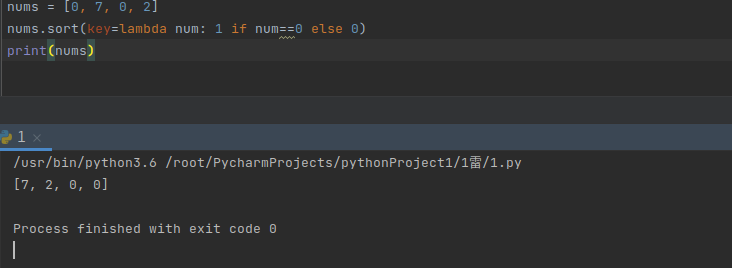## 二封装

1). 将内容封装到某处
2). 从某处调用被封装的内容
1). 通过对象直接调用被封装的内容： 对象.属性名
2). 通过self间接调用被封装的内容： self.属性名
3). 通过self间接调用被封装的内容： self.方法名()

``````# 类(Class)
class Cat:
def __init__(self, name, kind):  # 形参
"""
1. 构造方法，实例化对象时自动执行的方法
2. self是什么? self实质上是实例化的对象
3. 类方法中, python解释器会自动把对象作为参数传给self
"""
print('正在执行__init__构造方法')
print('self:', self)
# 属性：一般是名词，eg: name, age, gender.....
# 封装: self.name将对象和name属性封装/绑定
self.name = name
self.kind = kind
# 方法: 一般情况是动词, eg: create, delete, eating, run......
def eat(self):
print('cat %s like eating fish.....' %(self.name))

# 对象(Object):对类的实例化(具体化)
fentiao = Cat("粉条", "美短虎斑")
print(fentiao.name)
print(fentiao.kind)
fentiao.eat()
``````## 三 继承

``````class Student:
"""父类Student"""
def __init__(self, name, age):
self.name = name
self.age = age
def learning(self):
print(f'{self.name}正在学习')

class MathStudent(Student):
"""MathStudent的父类是Student"""
pass

# 实例化
m1 = MathStudent("粉条博士", 8)
print(m1.name)
print(m1.age)
m1.learning()           # 不报错，子类里没有，但父类有该方法
# m1.choice_course()   # 报错, 子类里没有，父类也没有的方法
``````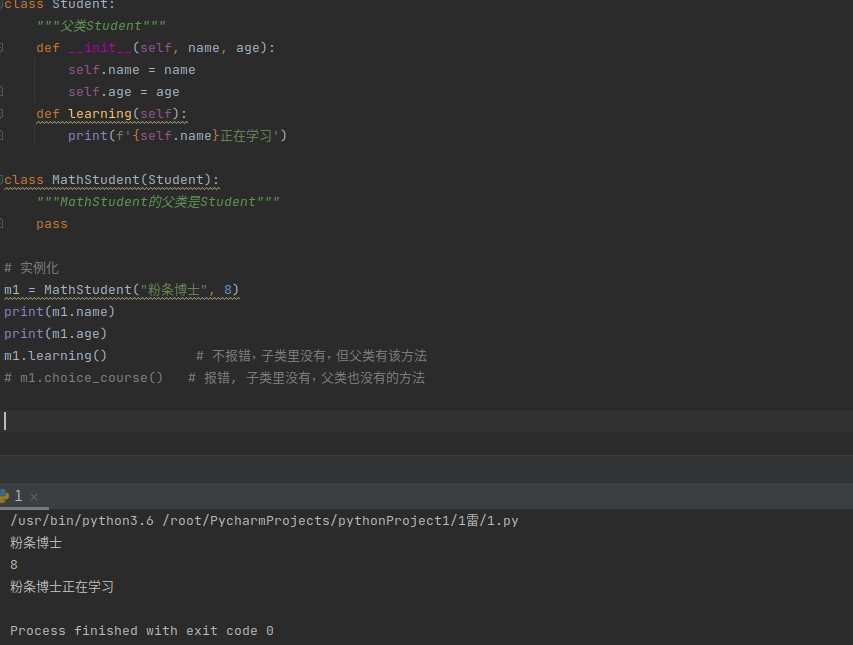``````class Student:
"""父类Student"""
def __init__(self, name, age):
self.name = name
self.age = age
def learning(self):
print(f'{self.name}正在学习')

def choice_course(self):
print('正在选课中'.center(50, '*'))

class MathStudent(Student):
"""MathStudent的父类是Student"""
def choice_course(self):
# 需求: 先执行父类的choice_course方法， 在个性化执行自己的方法。
# Student.choice_course(self)
# 解决方法1: 直接执行父类的方法，但不建议
# 解决方法2：通过super找到父类，再执行方法(建议且生产环境代码常用的方式)
super(MathStudent, self).choice_course()
info = """
课程表
1. 高等数学
2. 线性代数
3. 概率论
"""
print(info)

# 实例化
m1 = MathStudent("粉条博士", 8)
m1.choice_course()

s1 = Student("粉条博士", 8)
s1.choice_course()
``````

## 四 多继承

• 经典类多继承搜索顺序(深度优先算法):先深入继承树左侧查找，然后再返回，开始查找右侧。

• 新式类多继承搜索顺序(广度优先算法):先在水平方向查找，然后再向上查找。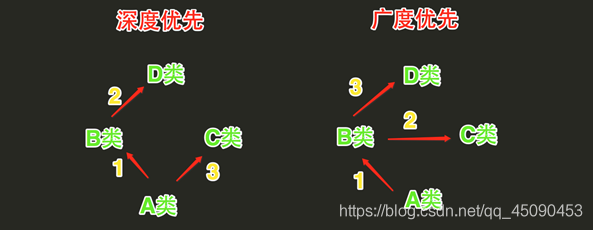``````"""

python3所有的类都属于新式类。新式类的继承算法是广度优先。

# 分析多继承的相关代码
>pip install djangorestframework
from rest_framework import viewsets
viewsets.ModelViewSet
"""

class D(object):
def hello(self):
print('D')
class C(D):
# def hello(self):
#     print('C')
pass
class B(D):
pass
# def hello(self):
#     print('B')
class A(B, C):
pass
# def hello(self):
#     print('A')
a = A()
a.hello()
``````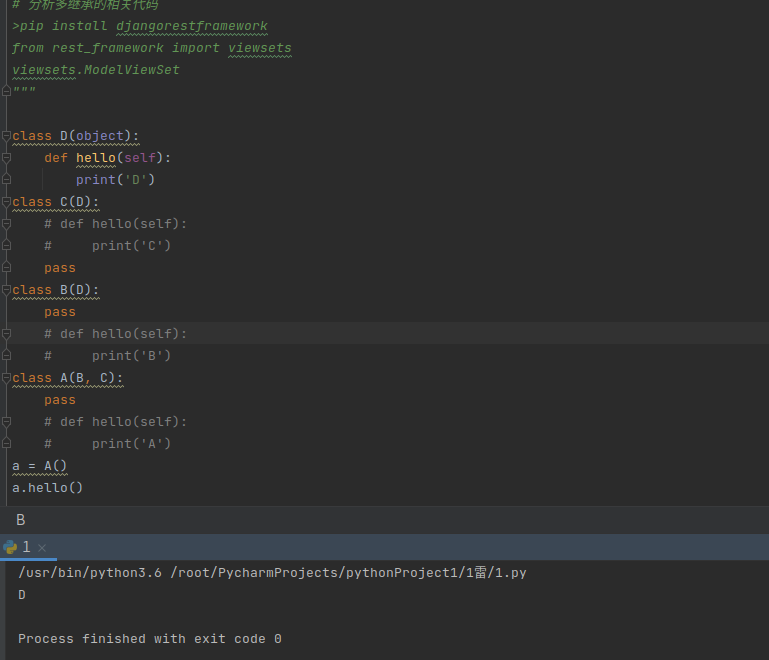## 五 私有属性与私有方法

确保了外部代码不能随意修改对象内部的状态,这样通过访问限制的保护,代码更加健壮。
如果又要允许外部代码修改属性怎么办?可以给类增加专门设置属性方法。 为什么大费周折?因为在方法中,可以对参数做检查,避免传入无效的参数

``````class Student:
"""父类Student"""
def __init__(self, name, age, score):
self.name = name
self.age = age
# 私有属性，以双下划线开头。
# 工作机制: 类的外部(包括子类)不能访问和操作，类的内部可以访问和操作。
self.__score = score

def learning(self):
print(f'{self.name}正在学习')

def get_score(self):
self.__modify_score()
return  self.__score

# 私有方法是以双下划线开头的方法,
#工作机制: 类的外部(包括子类)不能访问和操作，类的内部可以访问和操作。
def __modify_score(self):
self.__score += 20

class MathStudent(Student):
"""MathStudent的父类是Student"""
def get_score(self):
self.__modify_score()
return  self.__score

# 报错原因: 子类无法继承父类的私有属性和私有方法。
s1 = MathStudent('张三', 18, 100)
score = s1.get_score()
print(score)
``````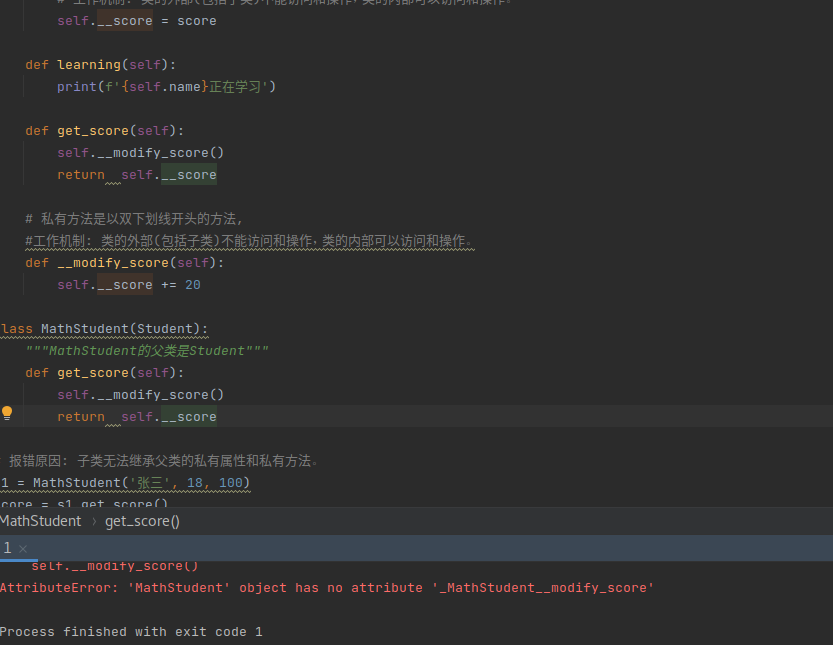## 六 多态

多态的好处就是，当我们需要传入更多的子类，只需要继承父类就可以了，而方法既可以直接不重写（即使用父类的），也可以重写一个特有的。这就是多态的意思。调用方只管调用，不管细节，而当我们新增一种的子类时，只要确保新方法编写正确，而不用管原来的代码。这就是著名的“开闭”原则：
对扩展开放（Open for extension）：允许子类重写方法函数
对修改封闭（Closed for modification）：不重写，直接继承父类方法函数

## 七 栈的封装

``````class Stack(object):
"""栈的封装[1, 2, 3, 4]"""

def __init__(self):
self.stack = []

def push(self, value):
"""入栈"""
self.stack.append(value)
print(f"入栈元素为{value}")

def pop(self):
"""出栈"""
if self.is_empty():
raise  Exception("栈为空")
item = self.stack.pop()
print(f"出栈元素为{item}")
return  item

def is_empty(self):
"""判断栈是否为空"""
return  len(self.stack) == 0

def top(self):
"""返回栈顶元素"""
if self.is_empty():
raise  Exception("栈为空")
return  self.stack[-1]

def __len__(self):
"""魔术方法, len(object)自动执行的方法"""
return  len(self.stack)

if __name__ == '__main__':
stack = Stack()
stack.push(1)
stack.push(2)
stack.push(3)
print(len(stack))  # 3
stack.pop()
print(stack.is_empty()) # False
print(stack.top())  # 2
``````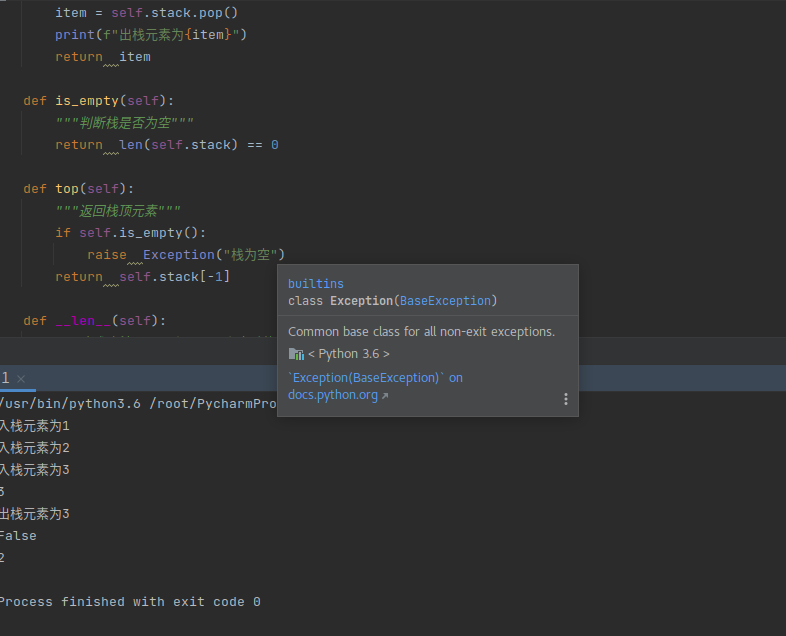## 八 队列的封装

``````class Queue(object):
"""
队列的封装
1. 列表的左侧队尾
2. 列表的右侧队头
"""
def __init__(self):
self.queue = []

def enqueue(self, value):
"""入队"""
self.queue.insert(0, value)
print("入队元素为:", value)

def dequeue(self):
"""出队"""
if self.is_empty():
raise  Exception("队列为空")
item = self.queue.pop()
print("出队元素:", item)
return  item

def __len__(self):
"""获取队列的长度"""
return  len(self.queue)

def first(self):
"""获取队头元素"""
if self.is_empty():
raise Exception("队列为空")
return  self.queue[-1]

def last(self):
"""获取队尾元素"""
if self.is_empty():
raise Exception("队列为空")
return  self.queue

def is_empty(self):
"""判断队列是否为空"""
return  len(self.queue) == 0

if __name__ == '__main__':
queue = Queue()
queue.enqueue(1)
queue.enqueue(2)
queue.enqueue(3)
print(queue.is_empty()) # False
queue.dequeue()  # 1出队， 队列只剩32
print(queue.first())  # 2
print(queue.last())  # 3
``````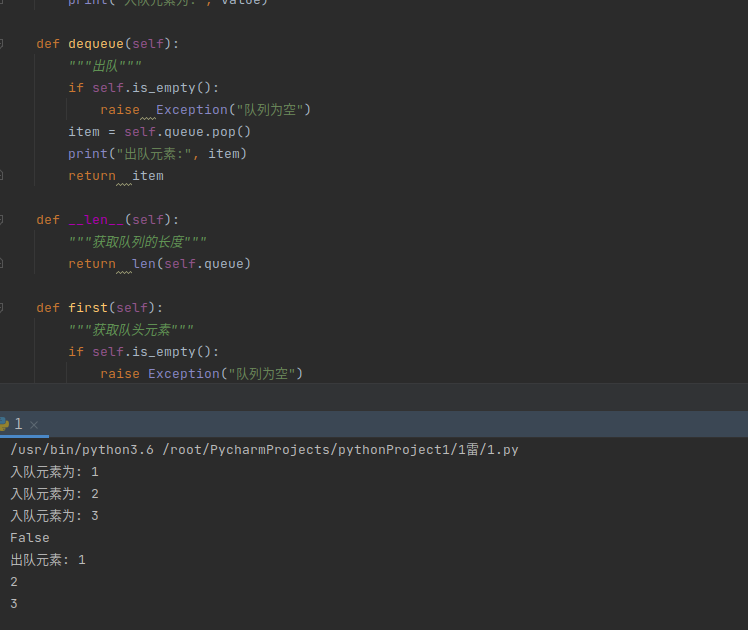## 九 二叉树的封装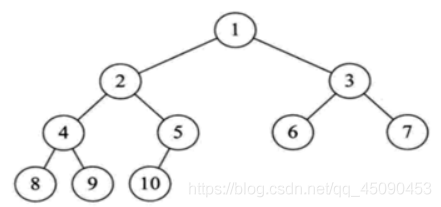``````

class Node(object):
"""节点类"""
def __init__(self, val=None, left=None, right=None):
self.val = val
self.left = left
self.right = right

class BinaryTree(object):
"""封装二叉树"""
def __init__(self, root):
self.root = root

def pre_travel(self, root):
"""先序遍历: 根左右"""
if (root != None):
print(root.val)
self.pre_travel(root.left)
self.pre_travel(root.right)

def in_travel(self, root):
"""中序遍历: 左根右"""
if (root != None):
self.in_travel(root.left)
print(root.val)
self.in_travel(root.right)

def last_travel(self, root):
"""后序遍历: 左右根"""
if (root != None):
self.last_travel(root.left)
self.last_travel(root.right)
print(root.val)

if __name__ == '__main__':
node1 = Node(1)
node2 = Node(2)
node3 = Node(3)
node4 = Node(4)
node5 = Node(5)
node6 = Node(6)
node7 = Node(7)
node8 = Node(8)
node9 = Node(9)
node10 = Node(10)

bt = BinaryTree(root=node1)
node1.left = node2
node1.right = node3
node2.left = node4
node2.right= node5
node3.left = node6
node3.right = node7
node4.left = node8
node4.right = node9
node5.left = node10

# 先序遍历
bt.pre_travel(node1)
print('--------')
# 中序遍历
bt.in_travel(node1)
print('--------')
# 后序遍历
bt.last_travel(node1)
``````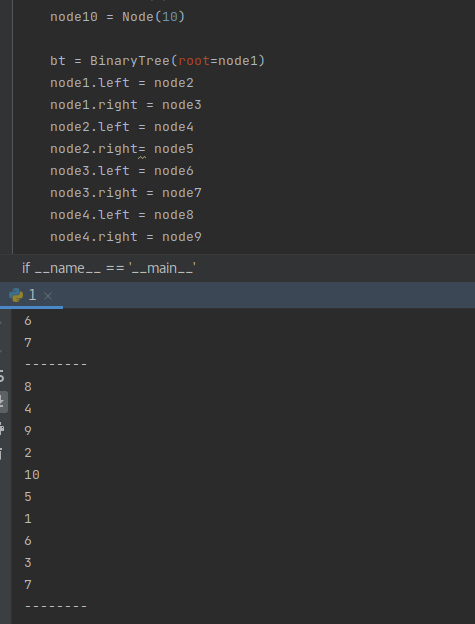## 十 高级特性

类方法是类对象所拥有的方法,需要用修饰器一般以@classmethod来标识其为类方法,
1). 对于类方法,第一个参数必须是类对象,作为第一个参数(cls是形参， 可以修改为其它变量名,但最好用’cls’了)
2). 能够通过实例对象和类对象去访问。
静态方法需要用修饰器一般以@staticmethod来标识其为静态方法,
1). 静态方法不需要多定义参数
2). 能够通过实例对象和类对象去访问。

``````"""

"""

class Student(object):
def __init__(self, name, age):
self.name = name
self.age = age

# 实例方法， python解释器会自动将对象/实例传入方法。
def get_age(self):
print('self:', self)
return  self.age

# 类方法:python解释器会自动将类传入方法。
@classmethod
def get_cls(cls):
print('cls:', cls)

# 静态方法:python解释器不会自动传入任何参数
@staticmethod
def get_info():
print("static method信息")

if __name__ == '__main__':
s = Student('张三', 18)
s.get_age()
s.get_cls()
s.get_info()
``````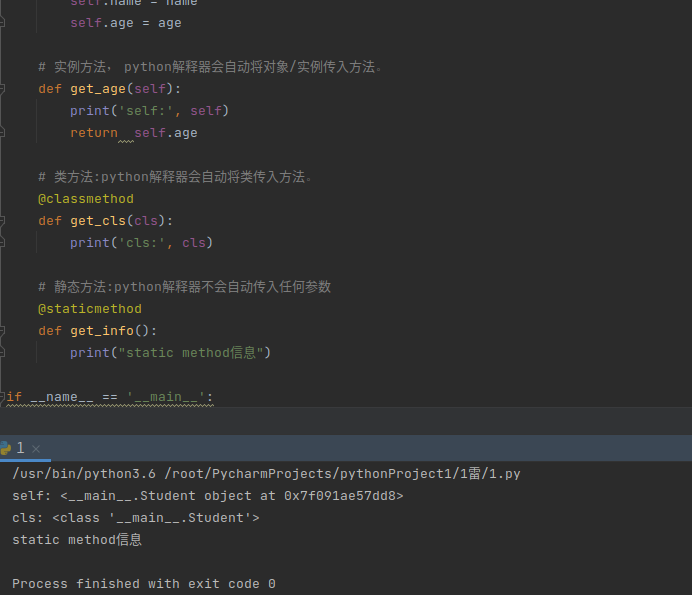``````class date(object):
# 正常的方法: 将对象作为参数传给self
def get_self(self):
print('self:', self)

# 类方法: 将类名作为参数传给cls
@classmethod
def get_cls(cls):
print('cls:', cls)

# 静态方法:不自动传递任何参数
@staticmethod
def get_static(name, age):
print("静态方法", name, age)

d = date()
d.get_self()
d.get_cls()
d.get_static("张三", 18)
``````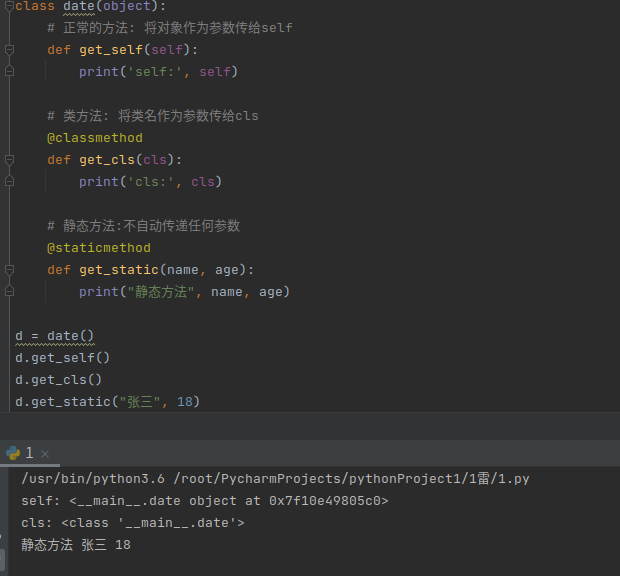## 十一 property类属性

property属性的定义和调用要注意一下几点：

定义时，在实例方法的基础上添加 @property 装饰器；并且仅有一个self参数
调用时，无需括号

property属性的有两种方式:

装饰器 即：在方法上应用装饰器
类属性 即：在类中定义值为property对象的类属性

经典类中的属性只有一种访问方式，其对应被 @property 修饰的方法
新式类中的属性有三种访问方式，并分别对应了三个被@property、@方法名.setter、@方法名.deleter修饰的方法

``````"""

- 根据用户请求的当前页和总数据条数计算出 m 和 n
- 根据m 和 n 去数据库中请求数据

from datetime import  datetime
"""

class Page(object):
"""
[user1, user2, user3......user100]
page=2, per_page=10
第一页: start=0 end=10
第二页: start=10 end=20
第三页: start=20 end=30
....
第page页: start=(page-1)*per_page end=page*per_page
"""
def __init__(self, page, per_page=10):
self.page = page
self.per_page = per_page

# 类属性: 将类方法变成类属性的过程。
@property
def start(self):
return (self.page-1) * self.per_page

@property
def end(self):
return  self.page * self.per_page

if __name__ == '__main__':
goods = ['good'+str(i+1) for i in range(100)]
page = Page(page=10, per_page=3)
print(goods[page.start:page.end])
``````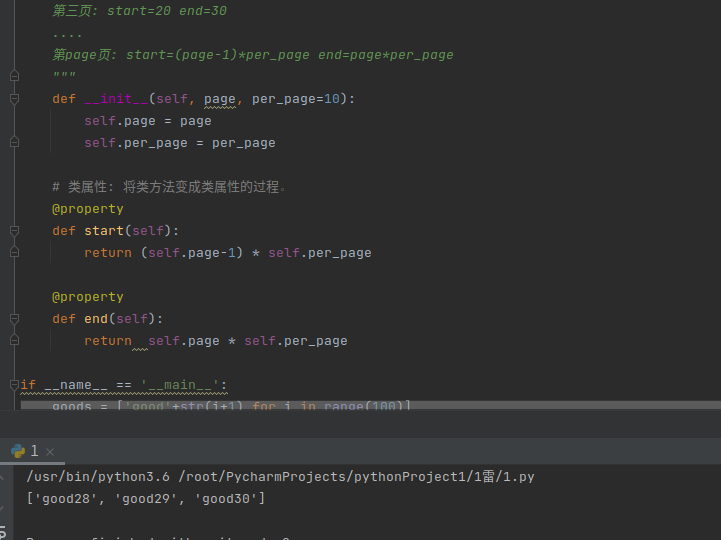``````class date(object):
def __init__(self, year, month, day):
# 私有属性
self.__year = year
self.__month = month
self.__day = day

# 将类方法object.year()转变成类属性object.year, 只是让代码更加简洁而已。
@property
def year(self):
return  self.__year

today = date(2021, 2, 27)
print(today.year)
``````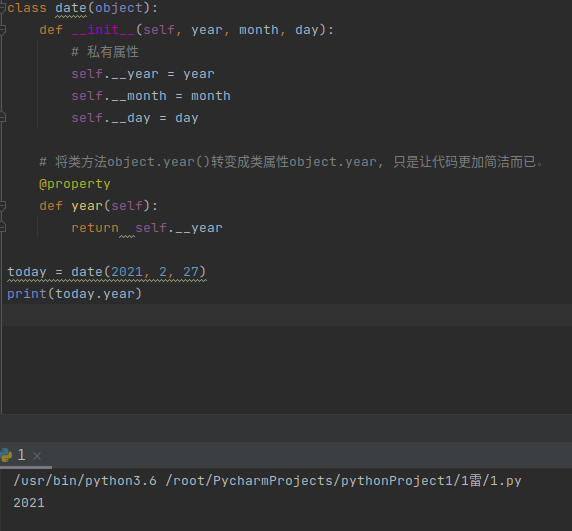## 十二 单例模式

``````"""

"""

class People(object):
pass

p1 = People()  # object
p2 = People()  # object
print(p1, p2)  # 每个对象的内存地址不同，肯定不是单例模式
``````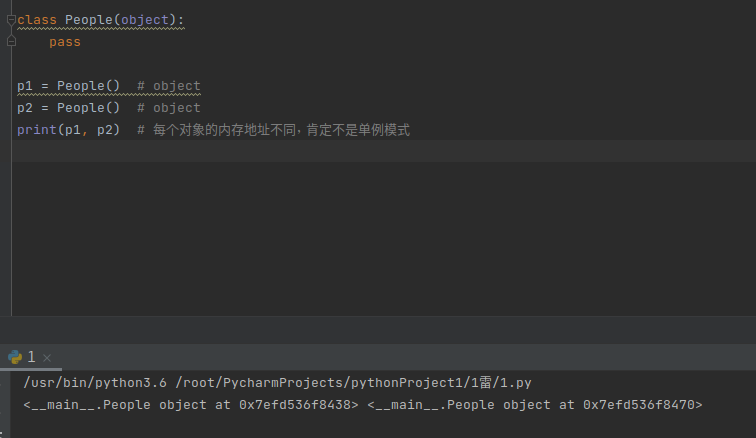基于装饰器实现单例模式

``````from functools import  wraps
def singleton(cls):
# 通过一个字典存储类和对象信息{"Class":"object"}
instances = {}
@wraps(cls)
def wrapper(*args, **kwargs):
# 为了保证单例模式， 判断该类是否已经实例化为对象
# 1. 如果有对象，直接返回存在的对象
# 2. 如果没有则实例化对象， 并存储类和对象到字典中， 最后返回对象
if instances.get(cls):
return instances.get(cls)
object = cls(*args, **kwargs)
instances[cls] = object
return  object
return  wrapper

@singleton
class People(object):
pass

p1 = People()
p2 = People()
print(p1, p2)
print(p1 is p2)  # 判断是否为单例模式(p1和p2内存地址是否相同)
``````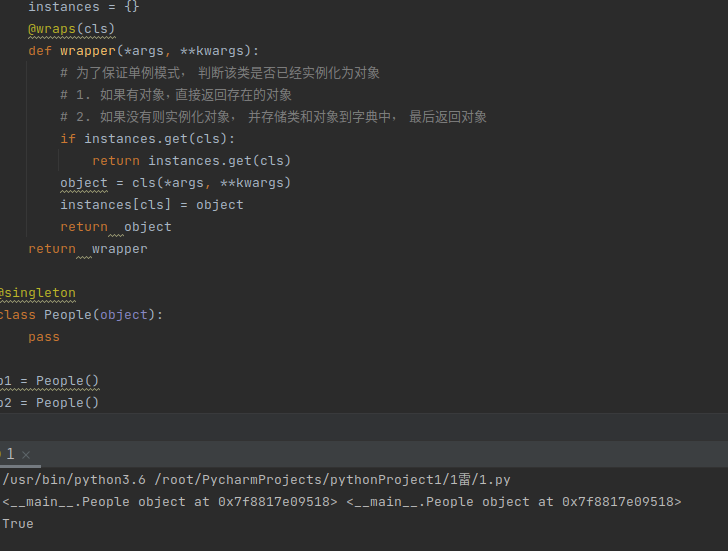## 十三 练习

实现商品信息类的封装

``````from datetime import datetime

class Medicine(object):
def __init__(self, name, price,PD,Exp):
self.name = name
self.price=price
self.PD=PD
self.Exp=Exp
def get_name(self):
return self.name
def get_GP(self):
start = datetime.strptime(self.PD,'%Y-%m-%d')
end=datetime.strptime(self.Exp,'%Y-%m-%d')
GP=end-start
return GP.days
def is_expire(self):
today=datetime.now()
oldday=datetime.strptime(self.Exp,'%Y-%m-%d')
if today>oldday:
return True
else:
return  False

if __name__ == '__main__':
medicineObj=Medicine('感冒胶囊',100,'2019-1-1','2019-3-1')
print('name:',medicineObj.get_name())
print('药品保质期为：',medicineObj.get_GP())
print('药品是否过期：','药品过期' if medicineObj.is_expire() else '药品未过期')
``````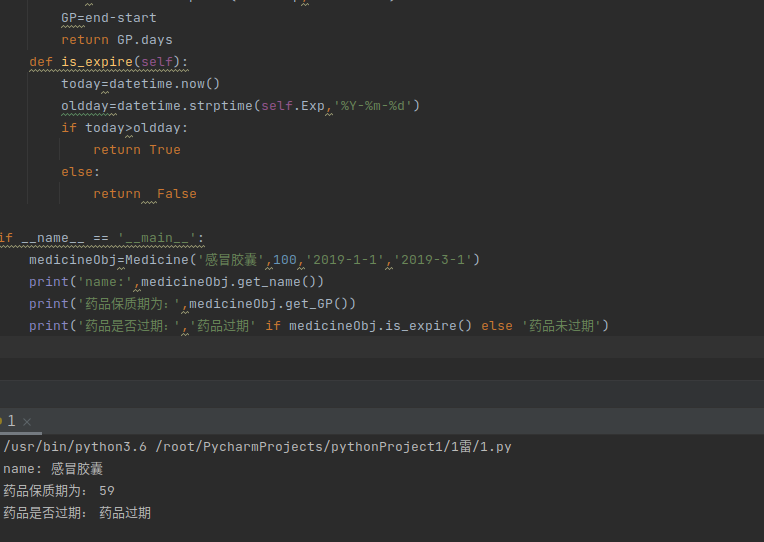LeetCode链表练习–两数相加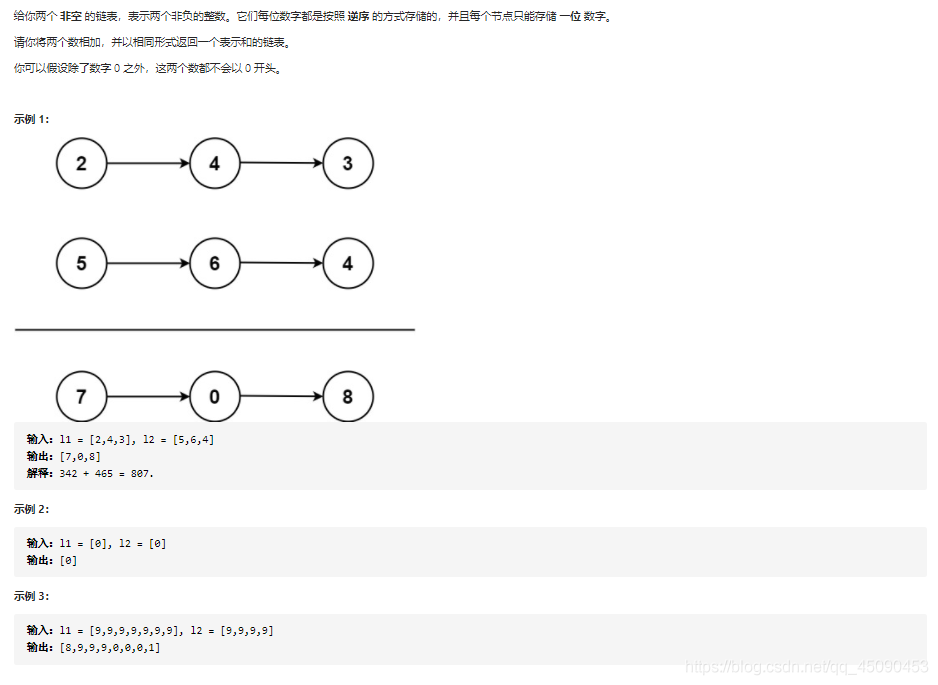``````

# 封装节点类
class ListNode:
def __init__(self, val=0, next=None):
self.val = val
self.next = next

"""遍历链表里面的每一个元素"""

def create_l1():
# l1 = 2,4,3
# l2 = 5, 6, 4
l1 = ListNode()
node1 = ListNode(val=2)
node2 = ListNode(val=4)
node3 = ListNode(val=3)
l1.next = node1
node1.next = node2
node2.next = node3
return  l1.next

def create_l2():
# l1 = 2,4,3
# l2 = 5, 6, 4
l2 = ListNode()
node1 = ListNode(val=5)
node2 = ListNode(val=6)
node3 = ListNode(val=4)
l2.next = node1
node1.next = node2
node2.next = node3
return  l2.next

def addTwoNumbers(l1: ListNode, l2: ListNode) -> ListNode:
res = 0
l3 = ListNode()
cur = l3
while(l1 or l2):
if(l1):
res += l1.val  # res=2
l1 = l1.next
if(l2):
res += l2.val # res=2+5=7
l2 = l2.next
# res=10, val=0, res=>val val=res%10
# res=14, val=4, 14%10=4
l3.next = ListNode(res%10)
l3 = l3.next
# res=10, 进位为1， 10//10=1
# res=14, 进位为1， 14//10=1
res  //= 10
if res == 1:
l3.next = ListNode(1)
return cur.next

if __name__ == '__main__':
l1 = create_l1()
l2 = create_l2()